### Home > PC > Chapter 1 > Lesson 1.1.3 > Problem1-36

1-36.
1. Let f(x) = 3x3 + 7 and g(x) = x2 − 1. Find and simplify the following function operations. Homework Help ✎

1. (fg)(x)

2.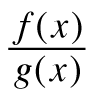3. fg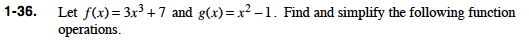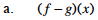What does the notation mean?

Subtract the g function from the f function.

3x3 + 7 − (x2 − 1)

3x3 + 7 − x2 + 1

3x3x2 + 8

$\frac{3x^{3} + 7}{x^{2} - 1}, x \not\equiv \pm 1$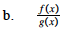Set up the division problem. Be sure you do not have a division by zero problem.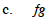Multiply the two functions.